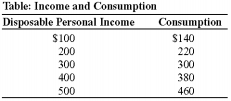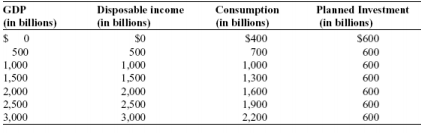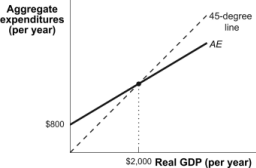×
Get Full Access to WM - ECON 102 - Class Notes - Week 5
Get Full Access to WM - ECON 102 - Class Notes - Week 5

×

WM / Economics / ECON 102 / if the mps rises, then the mpc will:

# if the mps rises, then the mpc will: Description

##### Description: Study Guide for Exam 3 with examples
10 Pages 138 Views 6 Unlocks
Reviews

## You will need to know it for the fourth exam, however?## What is the equilibrium level of income (Ye) and of GDP in this example?## (Table: The Economy of Albernia) What is the income–expenditure equilibrium GDP?1. The consumption function, including all related concepts, such as autonomous  consumption and the marginal propensity to consume. a. Consumption function­ equation showing how an individual household’s  consumer spending varies with the household’s We also discuss several other topics like anthony bordan
We also discuss several other topics like jovana pantovic
Don't forget about the age old question of How do children begin learning language?
Don't forget about the age old question of mth 271
We also discuss several other topics like deviance affirms cultural values and norms
We also discuss several other topics like What is a midpoint formula for a circle?
current disposable income i. c= a + MPC x yd  autonomous consumption is the “a” in the equation, it’s a constant,  individual household autonomous consumer spending, amount of spending a household would do if it had zero disposable income (we know this is  above 0 because people manage to borrow/ take \$ out of savings when  they have no income) ∙ “a” is also the y­intercept  marginal propensity to consume (MPC) is the percent of disposable  income a household spends versus saves  ∙ MPC= slope of function ∙ MPC+MPS =1 2. What causes the consumption function to shift and in what direction. 3. Things that can shift the aggregate consumption function: a. 1. Changes in expected future disposable income i. Example: Getting hired for a well­paying job would shift the aggregate  spending function up ii. Permanent income hypothesis: argument that spending depends more on  the income people expect to have long term than their current income  b. 2. Changes in aggregate wealth i. Example: 2 people with the same yd will spend differently if one has a  house and a lot of savings and the other does not­ increased wealth will  shift the consumption function up ii. Life­cycle hypothesis­ idea that consumers make decisions about spending  not based on their current disposable income, but on what they plan on  spending for their whole life (ie people put away money for retirement in  their 40s instead of spending every last bit of disposable income) 4. How to do calculations involving the consumption function and the MPC. a. Just know that MPC is the slope, a is the y­ intercept b. MPC will be (consumption spending­a)/ income i. Make sure to subtract a before calculating that ratio  c. Example of a practice problem:(Table: Income and Consumption) When disposable personal income is \$300, the MPC is:  C= A+ MPC(YD) 140=A +MPC(100), A = 140­ 100MPC 220= A + MPC(200) A= 220­ 200MPC 220­ 200MPC= 140­ 100 MPC 80=100MPC 0.8= MPC A) 0.80. B) 0.92. C) 0.95. D) 1.00. 5. The saving function, including all related concepts, such as the MPS. a. Negative A is the savings if Yd was 0. If income was zero then savings would be  negative (would spend out of savings or borrow) b. MPS= (1­MPC) c. MPS= ΔS/ ΔYd i. It is the ratio of savings to income, aka how much of your disposable  income you’re saving instead of spending 6. What causes the saving function to shift and in what direction. a. Saving function shifts as a result of changes in wealth and expected future  income, just like consumption function. Savings function always shifts in the  opposite direction of the consumption function b. As expected future income rises, saving function shifts down c. As wealth rises, saving function shifts down d. Also, as interest rates rise, savings function shifts up (again, opposite of what  consumption does) 7. How to do calculations involving the saving function and the MPS. a. Calculations with the savings function and MPS should be very similar to  consumption function problems, just with an opposite equation i. Remember: S= ­A+ (MPS)Yd b. You should also know: The wealth effect: As the overall price level rises, the  purchasing power of people’s financial wealth declines, so they cut back on  consumption in order to build­up their wealth again. i. The interest rate effect: As the overall price level rises, people need to  hold more money for transactions purposes, so demand for credit rises,  which causes interest rates to rise, which causes investment spending and  consumption spending to fall. 8. The aggregate expenditure function, its slope and where it is positioned relative to the  consumption function. a. AE=C+Ip b. AE= A+MPC*Y+Ipc. So slope= MPC i. It is positioned parallel and above the consumption function, since it is  equal to the consumption function + IP ( planned investment) 9. The AE­Y graph and how to use it to find changes in inventories. a. The AE­Y (aggregate expenditure – income) graph represents the relationship  between total spending and national income, at a given level of prices. ­ The income­ expenditure graph (99% sure this is the same thing) is the graph with the  aggregate expenditure function ( AE= A+ MPC(YD) +IP) and the 45 degree line together o Planned aggregate spending is determined by the equation C=A+MPC x YD o 45­degree line­ on a graph where Planned Aggregate Expenditure is on the y­axis  and Real GDP is on the x­axis, the 45 degree line graphs all the points where the  two could be equal (so it is just a line that starts at 0 and progresses with points at  (1,1), (2,2) and so on.  10. The equilibrium level of Y on the AE­Y graph and why national income and GDP tend to gravitate toward equilibrium. a. where planned AE and Real GDP cross unplanned investment=0 b. The place where these lines intersect is called the Keynesian Cross, which identifies the income­expenditure equilibrium  c. AE= A+ MPC (YD) +IP. Aggregate expenditure (AE) equals GDP in a closed  economy, but what this first equation does not account for is unplanned  investment. ( Investment= unplanned investment + planned investment)  i. Therefore, this equation only equals GDP when unplanned investment= 0 d. National income and GDP gravitate towards equilibrium because: i.  if GDP is higher than AEplanned, it means that there must have been  unplanned positive investment spending (firms overestimated sales and  produced too much). If GDP is lower than AEplanned, firms have  underestimated sales, and their inventory has been reduced ii. Firms will respond to unplanned positive investment spending by  investing less because they can see demand is less than they thought. They respond to unplanned negative investment spending because they see  demand is higher than they thought, and they can sell more. These  responses shift national income (Y) and GDP towards equilibrium  iii. 11. How to do calculations involving the AE equation, including how to calculate Ye,  unplanned investment, etc. a. Example problem: Table: The Economy of Albernia 12.13. 14. 12. (Table: The Economy of Albernia) What is the income–expenditure equilibrium GDP? Income expenditure equilibrium is where Yd=GDP=C A) \$1,000 billion B) \$1,500 billion C) \$2,000 billion D) \$2,500 billion Example number 2:  1. Consider the following levels of consumption spending, planned investment and national  income: Unplanned C   Ip Y     Investment (IU  ) Investment (I) 2060 200 2200 ­60 1402300 200 2500 0 200 2540 200 2800 60 260 Investment = Y­C. Income­ consumption equals investment. We also know this is true because  investment= savings, which as the same formula.  Unplanned investment= planned investment ­ Investment  IP­I = IU 2. Suppose that S=­400+.25Yd, Ip=\$500 and Y is \$3400. a. Calculate the change in inventories (unplanned investment) at this level of income. C= 400 + .75Yd, Ip=500, Y=3400 C= 400 + .75(3400) +500 C= 900 +2550=3450 Y­C= 3400­3450, so unplanned investment = ­50 b. What is the equilibrium level of income (Ye) and of GDP in this example? It will be where unplanned investment = 0 So where Y=C=GDP So set Y= to C C=400+ .75(C) +500  C= 900 +.75C .25 C=900 C= 900*4= 3600 So C=Y=GDP= 3600 at equilibrium 11. Planned investment, unplanned investment and Gross Private Domestic Investment (I). ­  Planned investment spending (IP) =investment spending firms intend to take in a given  business cycle (not always how it actually turns out) ­ Unplanned investment spending (IU)= investment spending that is deviates from the  expected spending. Can be positive or negative. If it’s positive, there was left over  product left over (more inventory than expected at the end of the year because demand  was lower than expected.) If it’s negative, then demand was higher than anticipated and  the firm had to dig into existing inventory to meet demand, leaving the firm with less  inventory than expected  ­ Gross Private Domestic Investment (I) is the I that goes into the GDP equation. I= IU + IP 12. What causes investment spending to change and in which direction. ­ ­ planned investment spending changes depending on on: interest rate, expected future  level of real GDP, and current level of production capacity o Interest rate If interest rates are high, products may not get purchased as often (think  new houses­ they are less likely to be sold if interest rates are high for  consumers.)   High interest rates also mean that businesses will borrow money up front  to invest less often, because their profits are increasingly less likely to be  able to pay off the loan as interest rates rise ∙ Or, if they don’t need to borrow money, they would rather lend out the money at a high interest rate than use it to invest o Expected future real GDP  If firms expect demand to increase, they will invest more to produce more   Accelerator principle­ a higher growth rate of real GDP leads to a higher  planned investment spending, but a lower growth rate of real GDP leads to a lower planned investment spending  o Current production capacity   Inventories­ stocks of goods held to satisfy future sales  Inventory investment­ value of the change in total inventories held in the  economy during a given period ∙ Can actually be negative­ if you have 100 cars in inventory, you  sell all the cars off the lot, and have to dig into the inventory stock, you may end the year with only 70 cars in inventory, therefore  negative 13. The spending multiplier in the context of the circular flow diagram. ­the spending multiplier is 1/ (1­MPC)  ­ multiplier­ the ratio of the total change in real GDP caused by an autonomous change in  aggregate spending to the size of that autonomous change  o how big were the ripples in comparison to the splash  o for example if there’s a  \$100 increase in investment, there will be an \$100  increase in Real GDP. This increase is called the autonomous change in aggregate spending (ΔAAE)  Multiplier= how much GDP changed as a result of ΔAAE: ΔAAE  ((MPC+MPC2+ MPC3+…)x\$100) : (1x\$100)  If ΔY= change in real GDP and ΔAAS= autonomous change in aggregate  spending, ∙  the multiplier= ΔY/ΔAAS or 1/(1­MPC) ­ In the circular flow diagram, firms produce goods and services equal in value to the total  income in the economy (Y.) this money flows to households, who save some and use the  rest to consume (we see this in the C arrow.) This consumption spending goes into AE  (total spending in the economy), which drives investment again. But wait! Firms also  contribute to AE with investment spending (that looping arrow thing.) When firms invest, it causes one big initial boost in GDP equal to the amount invested. However, it also  causes a ripple effect that goes around and around the circular flow. Say a firm invests  \$100. This causes a \$100 increase in GDP (we see this on the curve that travels to the left along the bottom there.) Once this additional \$100 hits households, people save a percentage of the \$100 (MPS) and spend a percentage (MPC). Lets say MPC is .6, so  they save \$40 and consume \$60. This \$60 flows down that little C arrow (top arrow,  pointing right,) and back into AE for firms to use to invest.  o So yay, now not only does the firm have its usual AE, it has \$60 additional from  its \$100 investment. Now it uses this \$60 to invest, that \$60 goes to GDP,  households get the \$60, they spend \$36 of it, that goes back into AE, etc etc the  cycle continues round and round. ­ ­ 15. The spending multiplier in the context of the AE­Y graph. a. ΔYe/ΔAAE = 1/(1­MPC) = 1/MPS, where AAE represents autonomous  expenditure in the economy, e.g. autonomous consumption. b. ΔY* (change to equilibrium income)= 1/(1­MPC) x ΔAAEplanned 16. How to do calculations involving the spending multiplier a. Understand that the multiplier =1/(1­MPC) = 1/MPS, so if marginal propensity to  save is 0.1 then the multiplier is 10(Figure: Aggregate Expenditures Curve II) Suppose that the consumption function in  this figure rises by \$100.The result would be an increase in the equilibrium level of real  GDP of: C=A+MPC(Y) C=800+MPC(2000) C=Y at equilibrium, so  2000=800 +2000MPC 1200= 2000MPC .6= MPC Multiplier= 1/(1­MPC) = 1/0.4= 2.5 So if consumption rises by \$100, real GDP will rise by 2.5(\$100) = \$250 A) \$100. B) \$200. C) \$250. D) \$50. 17. The saving­investment graph. a. Shows savings curve (S= ­A+(1­MPC)Yd ) intersecting investment, which we  assume is held equal b. At Ye (equilibrium income), savings= investment and the lines cross 18. Why the level of income where AE=GDP is the same level of income where S=Ip,  ignoring the macroeconomic impact of government and foreign trade. a. GDP =AEplanned+ Iunplanned i. So when GDP=AE, unplanned investment equals zero (at Ye) b. Savings funds investment  ( in a scenario without govt or foreign trade, private  savings are the only source of money for investment). Savings is equal to  investment at Ye.  i. At Ye , C+Ip = Y, so C+Ip = C+S,  1. (because in this simplified model C+S=Y) i. SO: Ip = S when AE=Y.  I.e., leakage = injection when the  macroeconomy is in equilibrium 19. Why investment is self­financing, according to Keynesian economics. a. without govt or foreign trade, private savings are the only source of money for  investment  b.  Rise in investment spending boosted AE, which boosted GDP, which boosted Y,  which boosted S, which funds investment again 20. Full analysis of the paradox of thrift. a. Same concept as before­ as we spend less, there’s less to invest, GDP falls,  income (Y) falls,  and then we end up having less money than if we had spent i. The multiplier effect applies here­ any reduction in investment as a result  of saving results in a (1/(1/MPS)*decrease in investment) decrease in GDP 21. The aggregate demand curve and the two reasons it slopes down.a. Aggregate demand curve­ negative relationship between real GDP demanded and  the overall price level (GDP deflator) because of:  i. 1. The wealth effect: as the overall level price level rises, the purchasing  power of people’ financial wealth declines, so they cut back on the  consumption in order to build up their wealth again ii. 2. The interest rate effect: As the overall price level rises, people need to  hold more money for transaction purposes, so demand for credit rises,  which causes interest rates to rise, which causes investment spending and  consumption spending to fall 22. Relationship between a movement along the AD curve and a shift of the AE line. a. I think he might have meant to say AD instead of AE, and this is referring to the  terminology differences between a shift and a movement along the line: i. A change in the aggregate quantity of goods and services, or real  GDP, demanded is characterized by movement along the aggregate  supply curve. ii. A change in aggregate demand is characterized by a change in the  aggregate quantity of goods and services demanded at every price level.   A shift of the AD curve b.  Otherwise, AE plots consumption against income and AD plots GDP against  prices. AE will shift up if there is a change in wealth or positive expectations for  the future. So presumably if the AE curve shifts up, the AD will shift up as well  (GDP demanded for the same goods at the same price will increase). But this is  one I’m not quite sure on 23. How a change in expectations, a change in wealth, fiscal policy and monetary policy shift the AD curve. a. Expectations of future income go up, curve shifts up b. Wealth goes up, curve goes up c. Fiscal policy­ a tax cut, increased transfers or govt spending would shift curve up  d. Monetary policy­ if interest rates rise, people will save more, curve will move  down 24. The short­run aggregate supply curve and why it slopes up. a. SRAS: Shows the relationship between aggregate output produced and the price  level, holding all other influences on firms’ production decisions constant (ceteris  paribus), and looking at a time frame within which workers’ nominal wages  cannot adjust to conditions in the labor market. b. Why SRAS slopes up: firm’s profit per unit of output= price of product­ cost of  uniti. If there’s a general increase in prices, ( and not enough time for income to  adjust, ) then the difference between price and cost of producing increases, firms make more money for each unit, and produce more 25. How a change in input (commodity) prices, a change in the nominal wage and a change  in productivity shift the SRAS curve. a. If Input prices rise, the curve shifts down b. If the nominal wage goes up, the curve shifts down c. If there’s a decrease in productivity, the curve shifts down 26. How to use the SRAS­AD graph to determine where the overall price level and real GDP  end­up. a. AD curve graphs Price level against real GDP demanded b. When SRAS and AD curves intersect, that will be the GDP supplied and price  level, because AD in this case is essentially a demand curve for the whole  economy and SRAS is a supply curve for the entire economy 27. How to analyze a change in expectations, a change in wealth, fiscal policy, monetary  policy, a change in input prices, a change in the nominal wage or a change in productivity on the SRAS­AD graph. a. Expectations of future income go up, AD curve shifts up­ both GDP and Price  will rise b. Wealth goes up, AD curve goes up­ both GDP and price rise c. Basically the same rules that were laid out for the individual curves apply here­ if  one curve shifts down, the price and GDP of the intercept will fall, and vice versa *Note: You will not be responsible for the long­run AS curve on the third exam.  You will need  to know it for the fourth exam, however.

Page ExpiredIt looks like your free minutes have expired! Lucky for you we have all the content you need, just sign up here